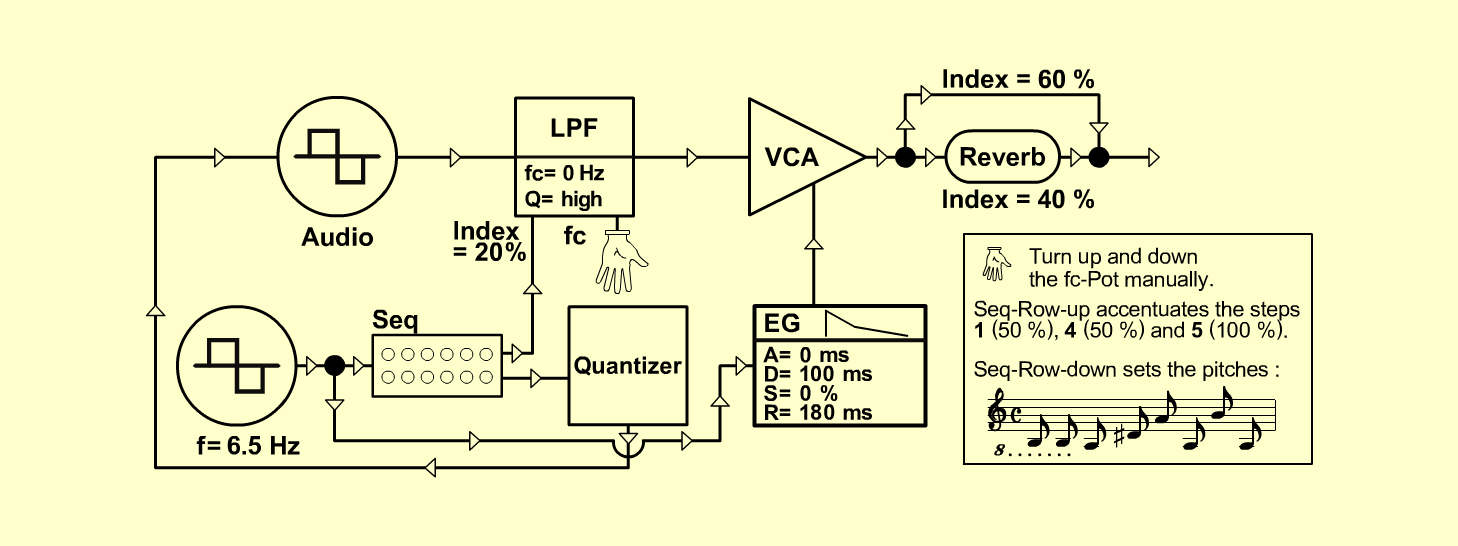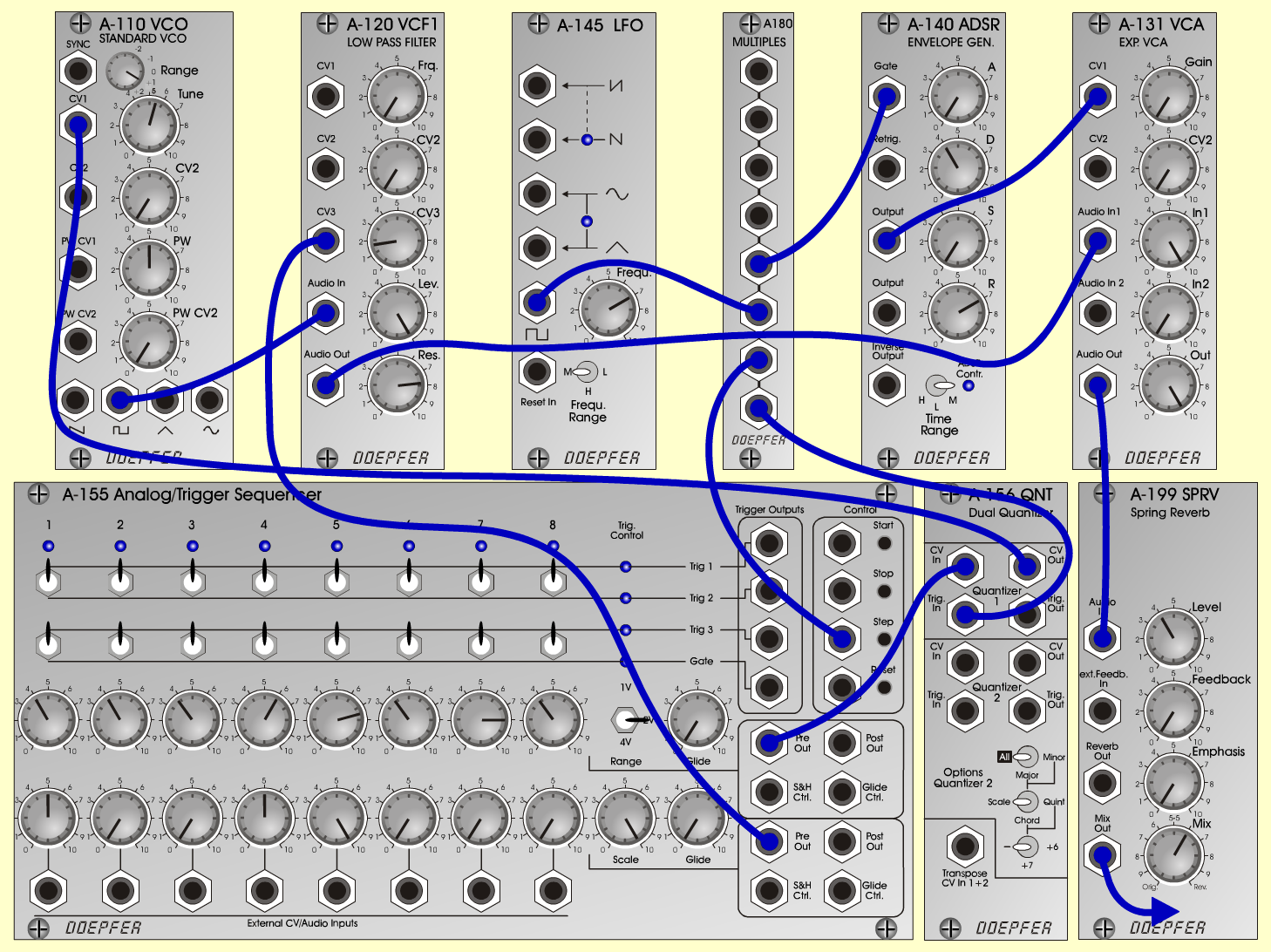PulsSweepTextureD o e p f e r A - 100 Connections: Settings: A-110 (Square) <=>  A-120 (Audio In)   A-120 (Audio Out) <=>  A-131 (Audio In)   A-145 (Square) <=>  A-180   A-180 <=> A-155 (Step/Clock)   A-180 <=> A-156/1 (Trig. In)   A-180 <=>  A-140 (Gate)   A-155 (Pre Out/1) <=>  A-156/1 (CV In)   A-155 (Pre Out/2) <=>  A-120 (CV 3)   A-156/1 (CV Out) <=>  A-110 (CV 1)   A-140 (Output) <=> A-131 (CV 1)   A-131 (Out) <=>  A-199 (Audio In)   A-199 (Mix Out)  <=> Amplifier A-110 (Range = +1, Tune = 5.5, PW = 5)   A-120 (Freq = 0, CV3 = 1.7, Lev = 10,    Res = 7.8)   A-145 (Frq = 7, Range = M)   A-140 (A = 0, D = 4, S = 0, R = 7, Range = M)   A-131 (Gain = 0, Audio In 1 = 10,    Audio Out = 10)   A-199 (Lev= 4, Feedb = 0, Emph = 0, Mix = 6)   A-155 (Row Up: 1= 4, 2= 4, 3= 3.8, 4= 6,    5= 7.5, 6= 3.8, 7= 8, 8= 3.8 , Range= 2 V,   Glide= 0/ Row Down: 1= 5, 2= 0, 3= 0, 4= 5,   5= 10, 6= 0, 7= 0, 8= 0, Scale= 10, Glide=0) Notes: Turn up and down the A-120-Freq-Pot    manually. Josef MuellerSound sample PulsSweep